# Sample Questions for Pattern Recognition Skills

1. Which of the figures can be used to continue the series given below?

2.•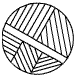•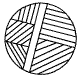•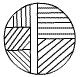•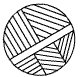•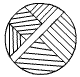Correct answer: C

Explanation: The base figure rotates at an angle of 450 in the anti-clockwise direction. Hence choice C is the perfect match.

3. Which of the figures, you think best fits the series below?

4.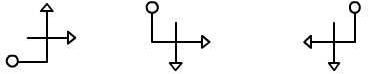•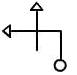•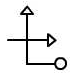•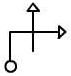•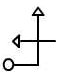Correct answer: A

Explanation: The base figure rotates at an angle of 900 in the clockwise direction. Hence choice A is the best fit.

5. Which of the figures can be used to continue the series given below?

6.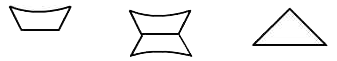•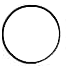•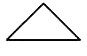•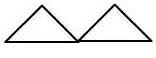•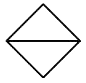Correct answer: D

Explanation: In the given series, a figure is followed by the combination of itself and its vertical inversion. Thus D. is the right choice.

7. If in a certain code language BOSTON can be written as SNSNSN, then how would you write CALIFORNIA in the same code language?

1. OAOAOA
2. LONLON
3. LOILOILOI
4. LONLONLON
Correct answer: 3. LOILOILOI

Explanation: The letters present in 3rd, 6th, 9th...etc (multiples of 3) positions are repeated thrice.

8. If NEW YORK can be encrypted as PGYAQTM, how can you code the word CHARLOTTE?

1. EICSNPVVG
2. EJCTNQVVG
3. EICTNPVVF
4. EJCSMPVVG
Correct answer: 2. EJCTNQVVG

Explanation: Each letter in the original word is forwarded two places (+2) in the alphabetical order, to get the encrypted word.

Take a PhD certified IQ test and find out your IQ in just 20 mins.

Go back to original article "Parts of IQ Test"

| | |

## Related Articles

Parts of IQ Test

An IQ test, tests your intelligence on different levels of thinking. It examines your following faculties:

1. Verbal Ability
2. Mathematical Ability
3. Spatial Reasoning Skills
4. spatial Skills
5. Classification Skills
6. Logical Reasoning Skills
7. Pattern Recognition Skills